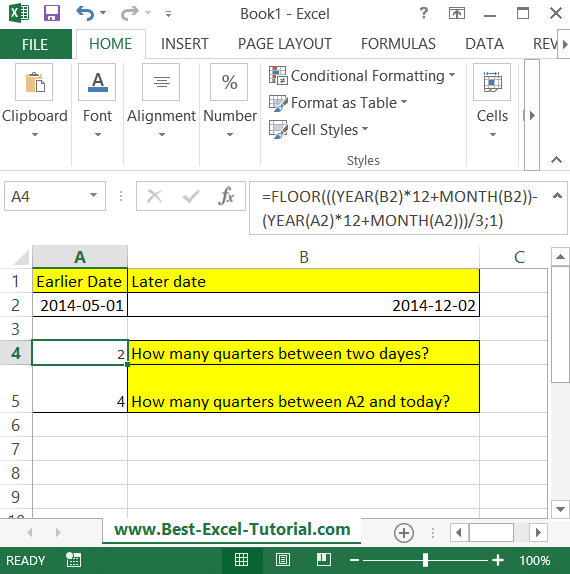#### Count of quarters between dates

In this Excel tutorial lesson, you will learn how to calculate the count of quarters between two dates using the Excel application.

## Quarters data analysis

Suppose you have two dates. You want to know how many quarters passed between these two dates. In cell B2 there is a later date. In cell A2 you typed an earlier date.## How many quarters between data formula

To calculate how many quarters are between these two days, just use the below formula.

=FLOOR(((YEAR(B2)*12+MONTH(B2))-(YEAR(A2)*12+MONTH(A2)))/3;1)

You can also calculate how many quarters there is between current day and some date (here in A2 cell).

=FLOOR(((YEAR(NOW())-YEAR(A2))*12+MONTH(NOW())-MONTH(A2))/3;1)

Results are rounded down.

You can count the number of quarters between two dates with the INT function using the following formula:

=INT((End_Date – Start_Date)/91)

This formula uses the INT function to round down the result to the nearest integer. The 91 in the formula represents the number of days in a quarter (91 days = 3 months).

For example, if the start date is 1/1/2021 and the end date is 4/1/2022, the result would be 4 quarters (1 + 1 + 1 + 1).

Note: This formula assumes that each quarter has exactly 91 days, which is not always the case. However, for most purposes, this formula provides a close approximation of the number of quarters between two dates.

You might also be interested in date duration calculator.